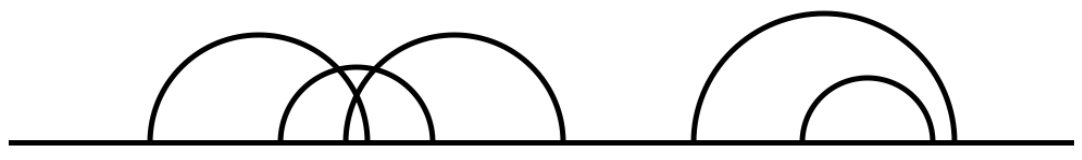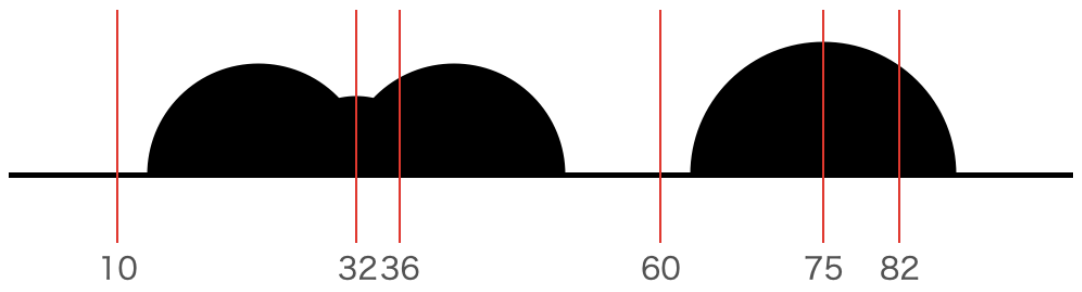시간 제한메모리 제한제출정답맞힌 사람정답 비율
2 초 512 MB0000.000%

## 문제

Mr. Mt. is eager to take photos of mountains all over the world. Today, Mr. Mt. visits one of the most interesting mountain ranges, the Alps of Curved Mountains. The shape of all the mountains of the Alps of Curved Mountains is, surprisingly, hemisphere.

Mr. Mt. just decided the place from where he will take photos of the mountains. The area covering the mountains is flat, horizontal ground, and the place he chose is far enough from the mountains. Hence the mountains look like two-dimensional semicircles in a row, where all the straight segments of the semicircles are aligned with a straight line. Some of them may be overlapped or completely covered by other semicircles.

Mr. Mt. is waiting for the sunset. After sunset, the mountains should be in shadow and cannot be distinguished. In that situation, Mr. Mt. is interested in how the photos will look like. You, his camera assistant, are also a great programmer. Given the coordinates and radiuses of all the semicircles, he asks you to write a program to calculate the heights of the 2D shadow at the positions he wants to know.

Figure F-1 illustrates the mountains of Sample 2. The first, second, third mountains are overlapped, and the fifth mountain is covered by the fourth mountain. Figure F-2 shows the mountains in shadow. For example, at position 82, there are the fourth and fifth mountains, and the shadow's height is the highest of them, the height of the fourth mountain.Figure F-1. Mountains of Sample 2Figure F-2. Mountains in shadow and positions of Sample 2

## 입력

The input consists of a single test case in the format below.

$N$

$c_1$ $r_1$

$\vdots$

$c_N$ $r_N$

$Q$

$x_1$

$\vdots$

$x_Q$

The first line contains a single integer $N$ ($1 \le N \le 10^5$), where $N$ is the number of mountains of the Alps of Curved Mountains. The $i$-th of the following $N$ lines contains two integers $c_i$ ($1 \le c_i \le 10^8$) and $r_i$ ($1 \le r_i \le 10^8$). $c_i$ is the position of the center of the $i$-th semicircle in a straight line, where "center" means the center of the circle made by reflecting the semicircle on the straight segment of the semicircle. $r_i$ is the radius of the $i$-th semicircle. The following line contains a single integer $Q$ ($1 \le Q \le 10^5$), where $Q$ is the number of the positions on a straight line Mr. Mt. is interested in. The $j$-th of the following $Q$ lines contains a single integer $x_j$ ($1 \le x_j \le 10^8$), where $x_j$ is the $j$-th position on a straight line Mr. Mt. is interested in.

## 출력

Output $Q$ lines, the $j$-th of which is the height of the shadow of the mountains at the $j$-th position Mr. Mt. is interested in. Absolute or relative errors less than $10^{-7}$ are permissible.

## 예제 입력 1

3
1 10
4 10
10 5
6
4
2
8
12
14
18


## 예제 출력 1

10.0000000000
9.9498743711
9.1651513899
6.0000000000
3.0000000000
0.0000000000


## 예제 입력 2

5
23 10
32 7
41 10
75 12
79 6
6
10
32
36
60
75
82


## 예제 출력 2

0.0000000000
7.0000000000
8.6602540378
0.0000000000
12.0000000000
9.7467943448


## 예제 입력 3

9
3 8
21 10
12 9
15 3
1 11
19 4
9 6
17 1
22 7
7
19
3
16
8
9
31
1


## 예제 출력 3

9.7979589711
10.8166538264
8.6602540378
8.4852813742
8.4852813742
0.0000000000
11.0000000000